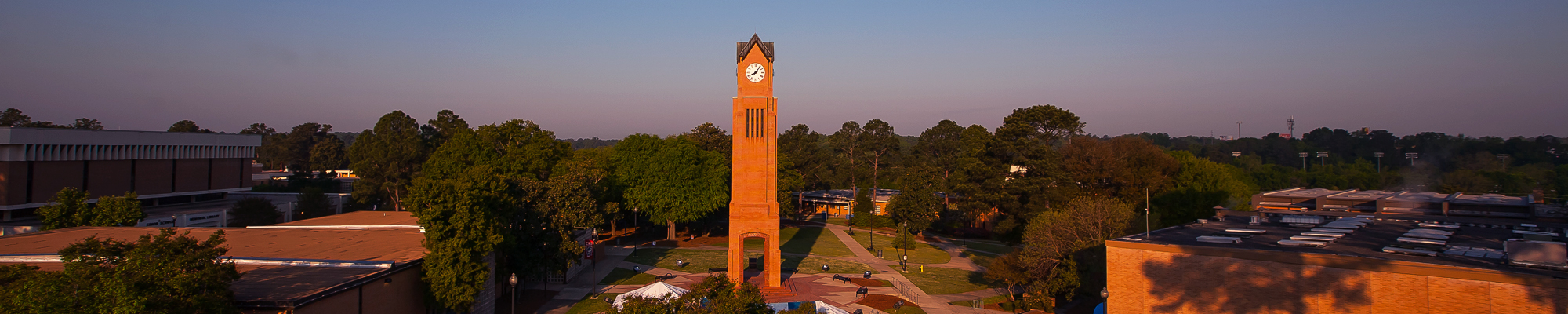## Math options

MATH 1001. Quantitative Skills and Reasoning (3-0-3) This course is for students needing practical, comprehensive instruction, with a focus on life applications, college level study abilities, and clear understanding of mathematics for additional coursework, careers and everyday living. NOTE: This course is an alternative in Area A of the General Education Core Curriculum and is not intended to supply sufficient algebraic background for students who intend to take College Algebra, Pre-calculus, or Calculus. Students may not receive credit for both MATH 1001 and MATH 1101.

MATH 1101. Introduction to Mathematical Modeling (3-0-3) This course is an introduction to mathematical modeling using graphical, numerical, symbolic, and verbal techniques to describe and explore real-world data and phenomena. Emphasis is on the use of elementary functions to investigate and analyze applied problems and questions, supported by the use of appropriate technology, and on effective communication of quantitative concepts and results. NOTE: This course is an alternative in Area A of the General Education Core Curriculum and is not intended to supply sufficient algebraic background for students who intend to take College Algebra, Pre-calculus, or Calculus. Students may not receive credit for both MATH 1001 and MATH 1101.(Course fee required.)

MATH 1111. College Algebra (3-0-3) Prerequisite: MATH 0195 or satisfactory Mathematics Placement Test score. This course is a functional approach to algebra that incorporates the use of appropriate technology. Emphasis will be placed on the study of functions, and their graphs, inequalities, and linear, quadratic, piece-wise defined, rational, polynomial, exponential, and logarithmic functions. Appropriate applications will be included. Course is designed to develop algebraic concepts to a level sufficient for the study of calculus. (Course fee required.)

MATH 1113. Pre-Calculus (4-0-4) Prerequisite: MATH 1111 with a grade of "C" or better or an appropriate math placement score. This course is designed to prepare students for calculus, physics, and related technical subjects. Topics include algebraic and transcendental functions and an intensive study of trigonometric functions.

MATH 1125. Applied Calculus (3-0-3) Prerequisite: MATH 1111 with a grade of "C" or better, MATH 1113 with a grade of "C" or better, or an appropriate math placement score. Introduction to limits and continuity, differential calculus of algebraic, exponential, and logarithmic functions and integration. Applications in the fields of the behavioral, biological, and managerial sciences are included. (Course fee required.)

MATH 1131. Calculus with Analytic Geometry 1 (4-0-4) Prerequisite: MATH 1113 with a grade of "C" or better or an appropriate math placement score. Topics include exponential and logarithmic functions, introduction to limits and derivatives, computation and application of derivatives, and the definite integral. (Course fee required.)